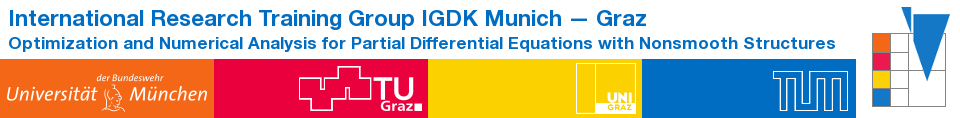IGDK1754QualificationProgramCompactCoursesCCFunke2016

# An introduction to solving partial differential equations in Python with FEniCS

### Simon Wolfgang Funke, PhD

#### Abstract

The FEniCS Project is a collection of open source software for the automated, efficient solution of partial differential equations. The software allows specifying finite element variational problems in close to mathematical form and, via automated code generation techniques, the automated assembly and solution of such. This is a powerful and exciting combination that enables rapid, reliable and fun development of efficient finite element models. In addition, the FEniCS extension dolfin-adjoint automates adjoint model implementation and provides an environment for rapidly solving PDE-constrained optimization problems.

This five-day course will consist of short lectures in combination with hands-on exercises aimed at novice and intermediate FEniCS users: starting from the very basics of the Finite Element method, to solving non-trivial, nonlinear, time-dependent PDEs, all the way to solving advanced, time-dependent PDE-constrained optimization problems.

Topics covered in the course include: solving linear static PDEs, solving nonlinear static PDEs, solving linear time-dependent PDEs, mixed problems, splitting methods, discontinuous Galerkin methods, adjoint methods and solving PDE-constrained optimization problems. Partial differential equations solved in the course include the Poisson equation, a nonlinear Poisson equation, the Stokes equations, nonlinear hyperelasticity (St. VenantKirchhoff), and the optimal control of the incompressible Navier-Stokes equations.